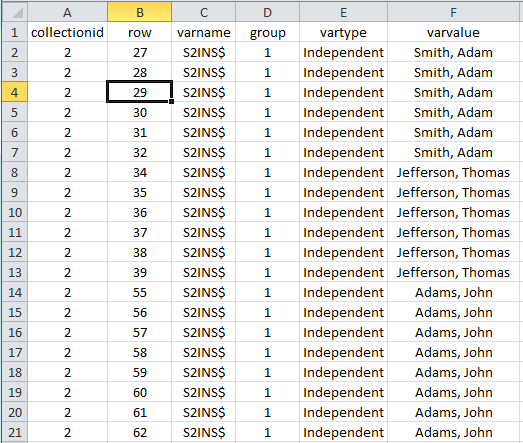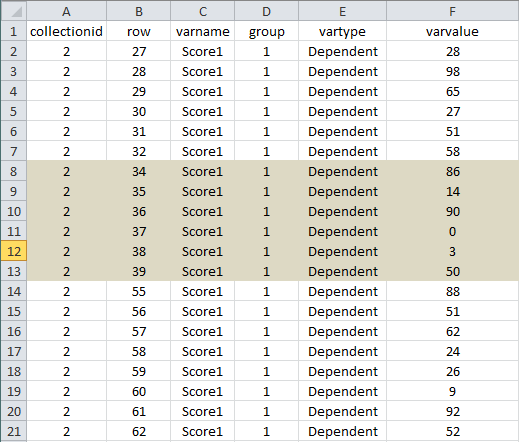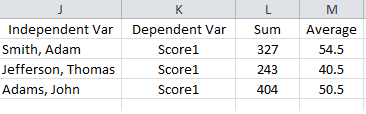# Subquery syntaxon
Hi Experts,

I have a table in SQL Server that represents data imported from a 2-d table with the following structure:

``````CREATE TABLE [dbo].[masterdata](
[collectionid] [int] NOT NULL,
[row] [int] NOT NULL,
[varname] [nvarchar](50) NOT NULL,
[group] [int] NOT NULL,
[vartype] [nvarchar](50) NULL,
[varvalue] [nvarchar](max) NULL,
CONSTRAINT [PK_masterdata] PRIMARY KEY CLUSTERED
(
[imparcid] ASC,
[row] ASC,
[varname] ASC
)WITH (PAD_INDEX  = OFF, STATISTICS_NORECOMPUTE  = OFF, IGNORE_DUP_KEY = OFF, ALLOW_ROW_LOCKS  = ON, ALLOW_PAGE_LOCKS  = ON) ON [PRIMARY]
) ON [PRIMARY]

GO
``````

Sample data would be:The challenge I can't seem to solve is I need to come up with a query that will provide the sum and average for certain other variables from the corresponding collectionid and row in the list based on the values for the varname listed.  So for example there is a dependent variable 'Score1' for which the data would be:The results of the query I need would be:I'm sure there is a way to form a subquery to do this but my SQL skills aren't yet up to it.

Thanks,
Deedub
Comment
Watch Question

Do more withEXPERT OFFICE® is a registered trademark of EXPERTS EXCHANGE®
SQL SERVER EXPERT

Commented:
what is the relation (KEY) between these 2 tables?
if just ROW ( not the best DB design)

try

select
m.[varvalue] 'Independed',
t.[varname] 'Depended',
sum(t.varvalue) SumScore,
AVG(t.varvalue) AvgScore

from masterdata m inner join table2 t on m.[row]=t.[row]
group by m.[varvalue] ,t.[varname]
Top Expert 2012

Commented:
Mine would be similar, just a different interpretation of the necessary table join...

SELECT m.varvalue as [Independent Var], v.varname as [Dependent Var], sum(v.varvalue) as [Sum], Avg(varvalue) as [Average]
FROM masterdata m INNER JOIN dependedvariabledata v
ON m.collectionid = v.collectionid and m.row = v.row and m.[group] = v.[group]
GROUP BY m.varvalue, v.varname

IF you want to filter for specific dependent variables, just add a WHERE clause to the statement after the join and before the grouping...

WHERE v.varvalue = 'Score1'

or for more than 1

WHERE v.varvalue in ('Score1','Score2','Score3')

Commented:
Actually it is only a single table, which is why I think I need to use subqueries.

Given the entries for a particular varname, I need the averages and sums for the varvalues of corresponding entries in the table based on that varname's collectionid and row for a different varname.

Commented:
The data in the table originally came from a series of variable-sized two-dimension tables (think of an Excel spreadsheets).  Each cell became it's own row in the table with the collectionid and group fields identifying the source spreadsheet, the row being the row number, and the varname being the column header.

The data structure is a given and I can't change it.

Through a query I need to come up with the average and sum of one of the columns (i.e varname = 'Score1') based on the values in another varname ('S2INS\$').  So in my example I have one of the varnames is S2INS\$ which is a person's name.  I need the average and sum for Score1 for each of the specific values of S2INS\$.

Back to the spreadsheet... it would be equivalent to subtotalling (giving the sum and average) the Score1 column based on the values in the S2INS\$ column.

I'm pretty sure this is doable with a subquery, but I'm not very good at them.

Hopefully (!) I'm clarifying what I need and not making it too confusing...
SQL SERVER EXPERT

Commented:
hmm, ok :
``````select
m.[varvalue] 'Independed',
t.[varname] 'Depended',
sum(t.varvalue) SumScore,
AVG(t.varvalue) AvgScore

from

(select varvalue,row,masterdata,varname where ISNUMERIC(varvalue)<> 1) m
inner join

(select varvalue,row,masterdata,varname where ISNUMERIC(varvalue)= 1) t
on m.[row]=t.[row]
group by m.[varvalue] ,t.[varname]
``````
SQL SERVER EXPERT
Commented:
also as per  Jared_S post

you can add  "   where t.[varname]= 'Score1'"

``````select
m.[varvalue] 'Independed',
t.[varname] 'Depended',
sum(t.varvalue) SumScore,
AVG(t.varvalue) AvgScore

from

(select varvalue,row,masterdata,varname where ISNUMERIC(varvalue)<> 1) m
inner join

(select varvalue,row,masterdata,varname where ISNUMERIC(varvalue)= 1) t
on m.[row]=t.[row]
where t.[varname]= 'Score1'
group by m.[varvalue] ,t.[varname]
``````
Information Technology Specialist

Commented:
select IndependentVar, DependentVar, sum, average from
(select t1.varvalue IndependentVar, t2.varname DependentVar,
sum(t2.varvalue) over (partition by t1.id, t1.varvalue order by t1.row) sum,
avg(t2.varvalue) over (partition by t1.id, t1.varvalue order by t1.row) avgerage,
row_number() over (partition by t1.id, t1.varvalue order by t1.row desc) rn
from tab1 t1, tab2 t2
where t1.id = t2.id and t1.row = t2.row) as x
where rn = 1;
Information Technology Specialist

Commented:
Sorry, I had to leave for a meeting and didn't refresh when I got back so I never saw all of these responses. Using the sum, avergage and group by that has been suggested should perform better than the windowing function that I showed.

Commented:
Thanks, that gets me where I need to be!

Do more with